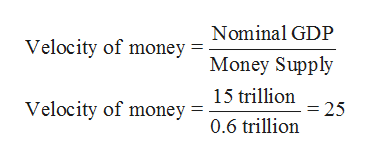# Suppose that this year's money supply is \$600 billion, nominal GDP is \$15 trillion, and real GDP is \$3 trillion.The price level is , and the velocity of money is . Suppose that velocity is constant and the economy's output of goods and services rises by 4 percent each year. Use this information to answer the questions that follow.If the Fed keeps the money supply constant, the price level will    , and nominal GDP will    . True or False: If the Fed wants to keep the price level stable instead, it should keep the money supply unchanged next year.True False  If the Fed wants an inflation rate of 9 percent instead, it should    the money supply by . (Hint: The quantity equation can be rewritten as the following percentage change formula: (Percentage Change in M)+(Percentage Change in V)=(Percentage Change in P)+(Percentage Change in Y)Percentage Change in M+Percentage Change in V=Percentage Change in P+Percentage Change in Y.)

Question
162 views
Suppose that this year's money supply is \$600 billion, nominal GDP is \$15 trillion, and real GDP is \$3 trillion.
The price level is

, and the velocity of money is

.

Suppose that velocity is constant and the economy's output of goods and services rises by 4 percent each year. Use this information to answer the questions that follow.
If the Fed keeps the money supply constant, the price level will    , and nominal GDP will    .

True or False: If the Fed wants to keep the price level stable instead, it should keep the money supply unchanged next year.
True

False

If the Fed wants an inflation rate of 9 percent instead, it should    the money supply by

. (Hint: The quantity equation can be rewritten as the following percentage change formula: (Percentage Change in M)+(Percentage Change in V)=(Percentage Change in P)+(Percentage Change in Y)Percentage Change in M+Percentage Change in V=Percentage Change in P+Percentage Change in Y.)

check_circle

Step 1

Since we answer only up to 3 sub-parts, we are answering the first three. If you want to get answered for the other sub-part as well then kindly post the question again.

It is given that the money supply is \$600 billion, nominal GDP is \$15 trillion and the real GDP is \$3 trillion.

a. The price level can be calculated by the formula given below:

Step 2

The velocity of money can be calculated by the following formula:help_outlineImage TranscriptioncloseNominal GDP Velocity of money Money Supply 15 trillion Velocity of money =25 0.6 trillion fullscreen
Step 3

b. If Fed keeps the money supply constant, the price level will fall and the nominal GDP will remain unchanged...

### Want to see the full answer?

See Solution

#### Want to see this answer and more?

Solutions are written by subject experts who are available 24/7. Questions are typically answered within 1 hour.*

See Solution
*Response times may vary by subject and question.
Tagged in